# Video abstract for "An Adaptive Homeostatic Algorithm for the Unsupervised Learning of Visual Features"

This video shows the results of unsupervised learning with different type of kezrnel normalization. This is to illustrate the results obtained in this paper on the An Adaptive Homeostatic Algorithm for the Unsupervised Learning of Visual Features which is now in press.

In :
```%matplotlib inline
```
In :
```%load_ext autoreload
```
%ls -l /tmp/cache_dir /tmp/2019-09-11*%ls -ltr /tmp/2019-09-11* %rm /tmp/cache_dir/*lock* %rm /tmp/2019-09-11*png%rm /tmp/2019-09-11_Perrinet19_None_*.png%rm /tmp/cache_dir/*
In :
```%%writefile /tmp/video_abstract.py
import os
import numpy as np
seed = 51
np.random.seed(seed)

from shl_scripts.shl_experiments import SHL
name = '2019-09-11_Perrinet19'

datapath = '/tmp/database'
opts = dict(datapath=datapath, verbose=True, cache_dir='/tmp/cache_dir', n_iter=2**5 + 1, seed=seed)
shl = SHL(**opts)
data = shl.get_data(matname=f'{name}_data')

homeo_methods = ['None', 'OLS', 'HEH', 'HAP']
N_step = 18

d_new, d_old = 1, 1
fibo = [d_old]
for i_step in range(N_step+1):
fibo.append(d_new)
d_new, d_old = d_new + d_old, d_new
print('Fibonacci=', fibo)

from shl_scripts import touch
#from shl_scripts.shl_experiments import SHL_set
for homeo_method in homeo_methods:
print(f'=> homeo_method={homeo_method}')
flockname = os.path.join(shl.cache_dir, f'{name}_{homeo_method}_lock')
if not os.path.isfile(flockname):
touch(flockname)
dictionary, P_cum = None, None
opts_ = opts.copy()
opts_.update(homeo_method=homeo_method, n_iter=1,)
shl = SHL(**opts_)
for i_step in range(N_step):
print(f'==> i_iter={i_step} / n_iter={fibo[i_step+1]}')
shl.n_iter = fibo[i_step]
dico = shl.learn_dico(data=data, dictionary=dictionary, P_cum=P_cum, matname=f'{name}_{homeo_method}_{i_step}')
dictionary = dico.dictionary
P_cum = dico.P_cum

if os.path.isfile(flockname): os.remove(flockname)

```
```Overwriting /tmp/video_abstract.py
```
In :
```%run  /tmp/video_abstract.py
```
```Extracting data..loading the data called : /tmp/cache_dir/2019-09-11_Perrinet19_data_data
Data is of shape : (65520, 441) - done in 0.15s.
Fibonacci= [1, 1, 2, 3, 5, 8, 13, 21, 34, 55, 89, 144, 233, 377, 610, 987, 1597, 2584, 4181, 6765]
=> homeo_method=None
==> i_iter=0 / n_iter=1
==> i_iter=1 / n_iter=2
==> i_iter=2 / n_iter=3
==> i_iter=3 / n_iter=5
==> i_iter=4 / n_iter=8
==> i_iter=5 / n_iter=13
==> i_iter=6 / n_iter=21
==> i_iter=7 / n_iter=34
==> i_iter=8 / n_iter=55
==> i_iter=9 / n_iter=89
==> i_iter=10 / n_iter=144
==> i_iter=11 / n_iter=233
==> i_iter=12 / n_iter=377
==> i_iter=13 / n_iter=610
==> i_iter=14 / n_iter=987
==> i_iter=15 / n_iter=1597
==> i_iter=16 / n_iter=2584
==> i_iter=17 / n_iter=4181
=> homeo_method=OLS
==> i_iter=0 / n_iter=1
==> i_iter=1 / n_iter=2
==> i_iter=2 / n_iter=3
==> i_iter=3 / n_iter=5
==> i_iter=4 / n_iter=8
==> i_iter=5 / n_iter=13
==> i_iter=6 / n_iter=21
==> i_iter=7 / n_iter=34
==> i_iter=8 / n_iter=55
==> i_iter=9 / n_iter=89
==> i_iter=10 / n_iter=144
==> i_iter=11 / n_iter=233
==> i_iter=12 / n_iter=377
==> i_iter=13 / n_iter=610
==> i_iter=14 / n_iter=987
==> i_iter=15 / n_iter=1597
==> i_iter=16 / n_iter=2584
==> i_iter=17 / n_iter=4181
=> homeo_method=HEH
==> i_iter=0 / n_iter=1
==> i_iter=1 / n_iter=2
==> i_iter=2 / n_iter=3
==> i_iter=3 / n_iter=5
==> i_iter=4 / n_iter=8
==> i_iter=5 / n_iter=13
==> i_iter=6 / n_iter=21
==> i_iter=7 / n_iter=34
==> i_iter=8 / n_iter=55
==> i_iter=9 / n_iter=89
==> i_iter=10 / n_iter=144
==> i_iter=11 / n_iter=233
==> i_iter=12 / n_iter=377
==> i_iter=13 / n_iter=610
==> i_iter=14 / n_iter=987
==> i_iter=15 / n_iter=1597
==> i_iter=16 / n_iter=2584
==> i_iter=17 / n_iter=4181
=> homeo_method=HAP
==> i_iter=0 / n_iter=1
==> i_iter=1 / n_iter=2
==> i_iter=2 / n_iter=3
==> i_iter=3 / n_iter=5
==> i_iter=4 / n_iter=8
==> i_iter=5 / n_iter=13
==> i_iter=6 / n_iter=21
==> i_iter=7 / n_iter=34
==> i_iter=8 / n_iter=55
==> i_iter=9 / n_iter=89
==> i_iter=10 / n_iter=144
==> i_iter=11 / n_iter=233
==> i_iter=12 / n_iter=377
==> i_iter=13 / n_iter=610
==> i_iter=14 / n_iter=987
==> i_iter=15 / n_iter=1597
==> i_iter=16 / n_iter=2584
==> i_iter=17 / n_iter=4181
```
In :
```phi = (np.sqrt(5) + 1. ) /2
fig_width = 15
dpi_export = 300
#dpi_export = 0
colors = ['black', 'orange', 'blue', 'red']

import matplotlib.pyplot as plt
def plot_dico(dico, color, dim_graph = (8, 8)):
subplotpars = dict(left=0.05, right=.95, bottom=0.05, top=.95, wspace=0.05, hspace=0.05,)
fig, axs = plt.subplots(1, 2, figsize=(fig_width, fig_width/phi), gridspec_kw=subplotpars)
for ax in axs:
ax.axis(c='b', lw=2, axisbg='w')
ax.set_facecolor('w')

from shl_scripts import show_dico
fig, axs = show_dico(shl, dico, data=data, dim_graph=dim_graph, fig=fig, ax=axs, order=False, seed=51)

if False:
from shl_scripts import plot_P_cum
fig, axs = plot_P_cum(dico.P_cum, ymin=0.6, ymax=1.001,
title=None, suptitle=None, ylabel='non-linear functions',
verbose=False, n_yticks=21, alpha=.02, c=color, fig=fig, ax=axs)
axs.plot(, , lw=1, color=color, label=homeo_method, alpha=.6)

else:
from shl_scripts import plot_proba_histogram
coding = shl.code(data, dico)
fig, axs = plot_proba_histogram(coding, fig=fig, ax=axs, lw=2., alpha=1., color='gray')
axs.plot(, , lw=1, color=color, label=homeo_method, alpha=.6)
# ax.set_ylabel(homeo_method)

#ax.text(-8, 7*dim_graph, homeo_method, fontsize=12, color='k', rotation=90)#, backgroundcolor='white'
#axs.legend(loc='lower right')
# pos : [left, bottom, width, height] =    The new position of the in `.Figure` coordinates.
axs.set_position([0.0, 0.0, .618, 1.0])
axs.set_position([0.618+0.05, 0.0+0.05, .382-0.07, 1.0-0.052])

return fig, axs

for homeo_method, color in zip(homeo_methods, colors):
print(f'=> homeo_method={homeo_method}')
flockname = os.path.join(shl.cache_dir, f'{name}_{homeo_method}_lock')
if not os.path.isfile(flockname):
for i_step in range(N_step):
dico = shl.learn_dico(data=data, matname=f'{name}_{homeo_method}_{i_step}', list_figures=[])

print(f'==> i_iter={i_step} / n_iter={fibo[i_step+1]}')
figname = f'/tmp/{name}_{homeo_method}_{i_step}.png'
if not os.path.isfile(figname):
fig, axs = plot_dico(dico, color)
axs.set_xlim(0., 1.9)
axs.text(0.1, 32, f'homeo_method={homeo_method}', fontsize=24, color=color, backgroundcolor='white')
axs.text(0.1, 92, f'n_iter={fibo[i_step+1]:4d}', fontsize=24, color='k', backgroundcolor='white')
plt.show()
if dpi_export > 0 : fig.savefig(figname, dpi=dpi_export, bbox_inches='tight')

```
```=> homeo_method=None
==> i_iter=0 / n_iter=1
Coding data with algorithm  mp ```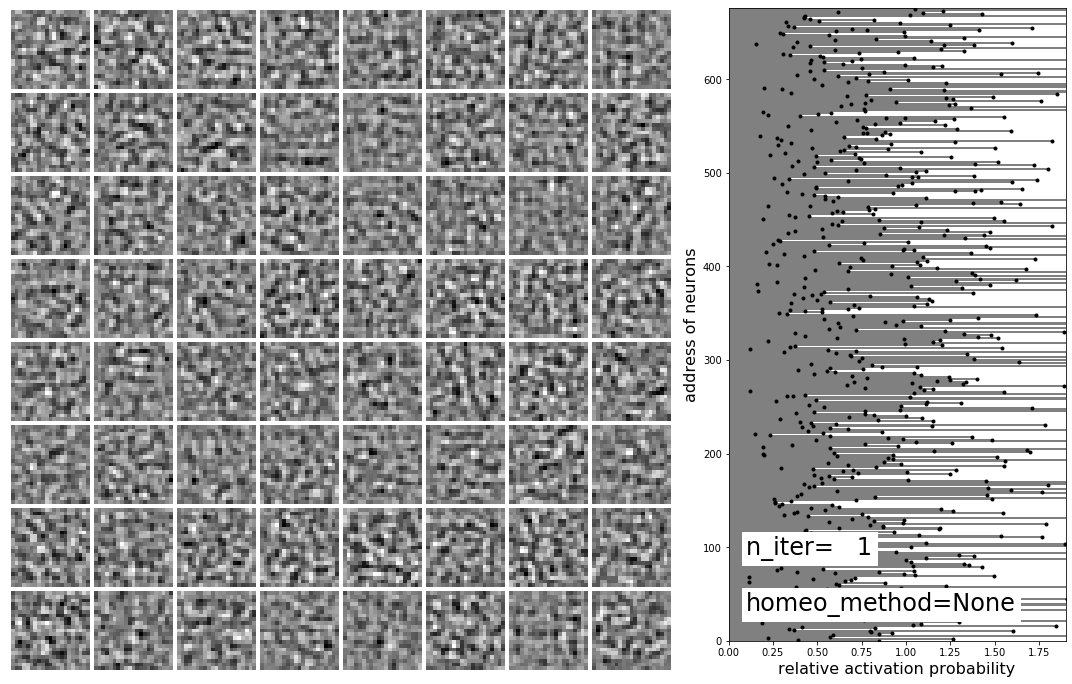```loading the dico called : /tmp/cache_dir/2019-09-11_Perrinet19_None_1_dico.pkl
==> i_iter=1 / n_iter=2
Coding data with algorithm  mp ```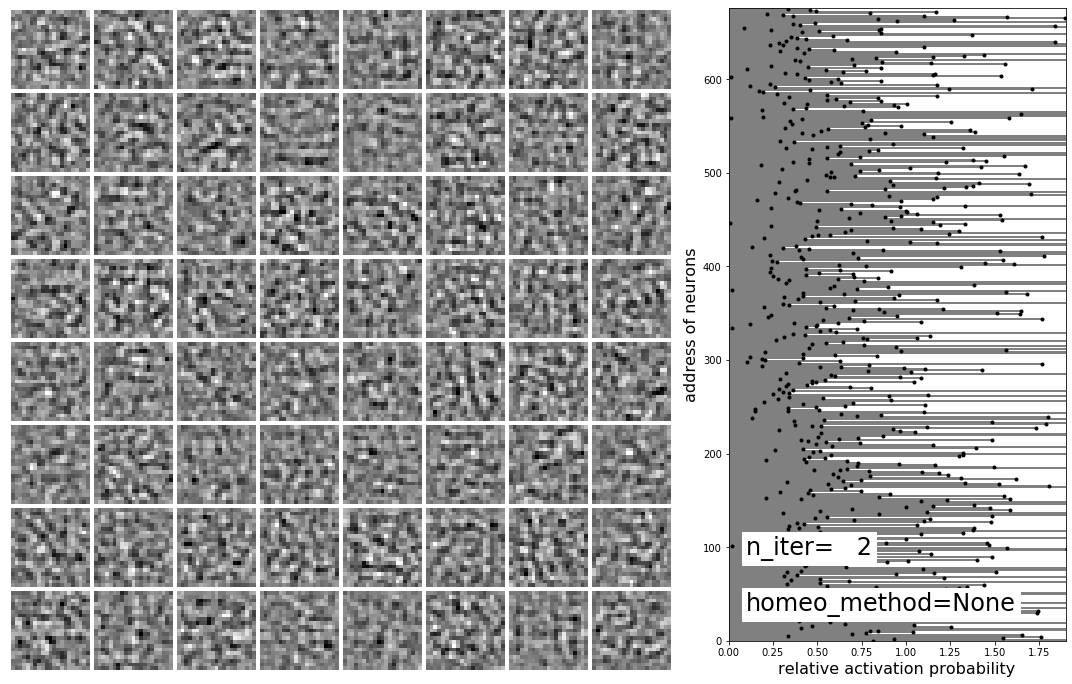```loading the dico called : /tmp/cache_dir/2019-09-11_Perrinet19_None_2_dico.pkl
==> i_iter=2 / n_iter=3
Coding data with algorithm  mp ```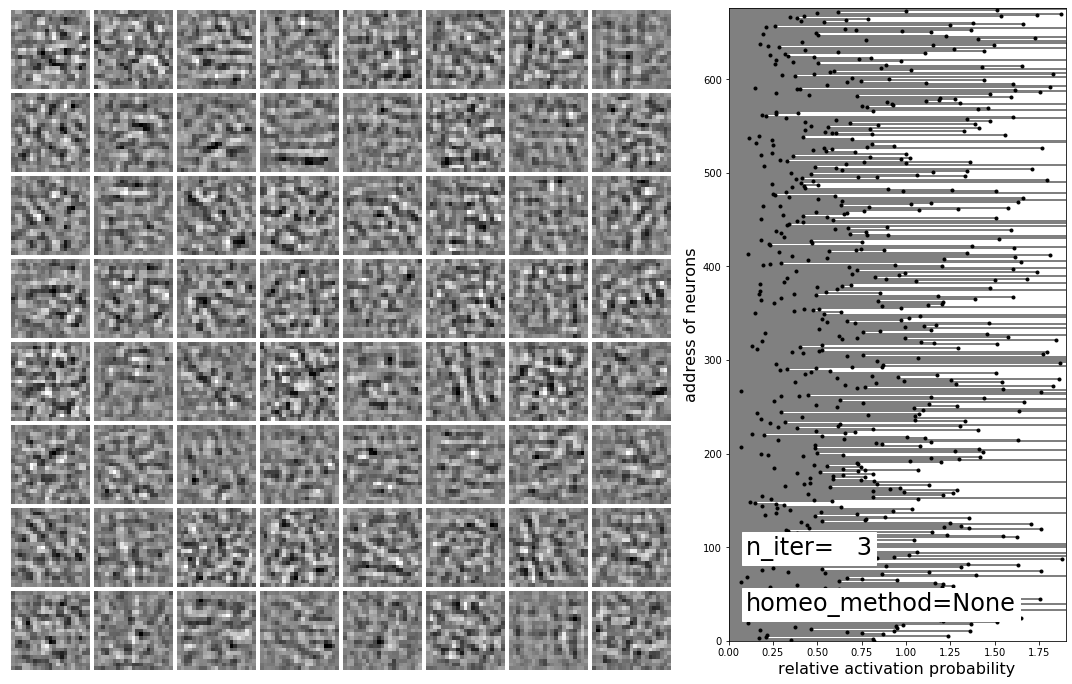```loading the dico called : /tmp/cache_dir/2019-09-11_Perrinet19_None_3_dico.pkl
==> i_iter=3 / n_iter=5
Coding data with algorithm  mp ```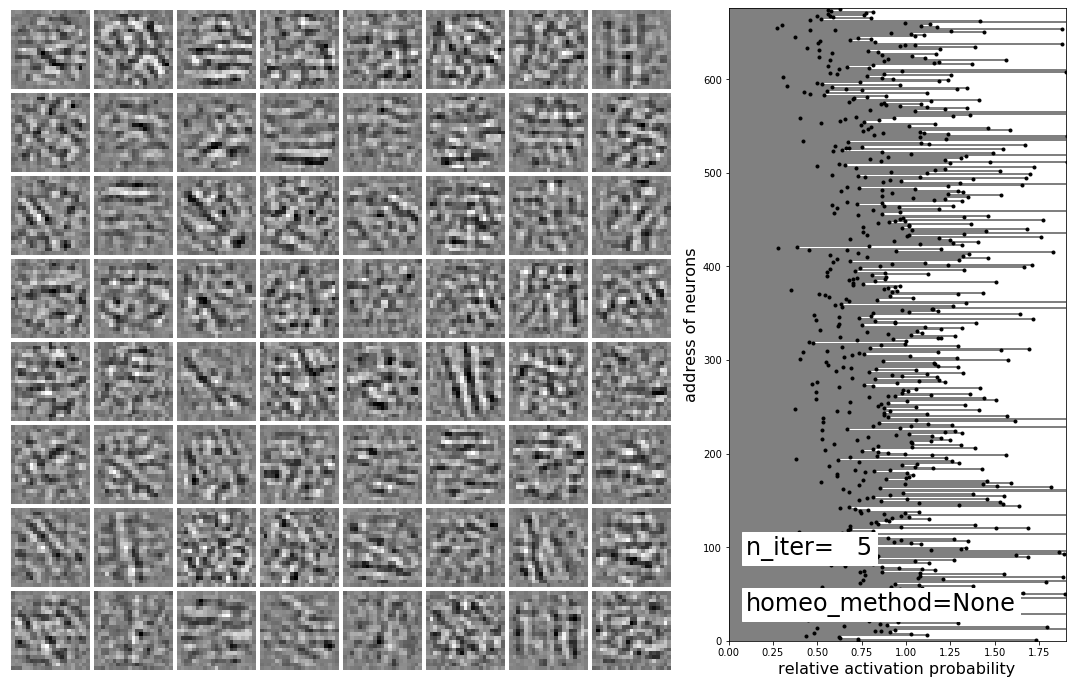```loading the dico called : /tmp/cache_dir/2019-09-11_Perrinet19_None_4_dico.pkl
==> i_iter=4 / n_iter=8
Coding data with algorithm  mp ```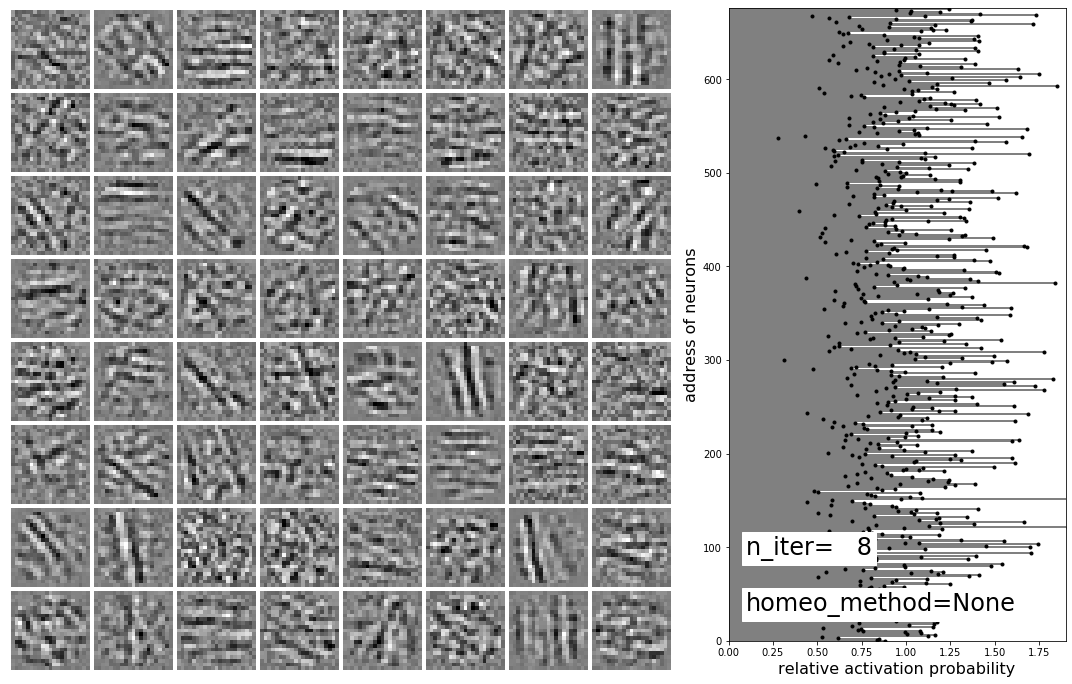```loading the dico called : /tmp/cache_dir/2019-09-11_Perrinet19_None_5_dico.pkl
==> i_iter=5 / n_iter=13
Coding data with algorithm  mp ```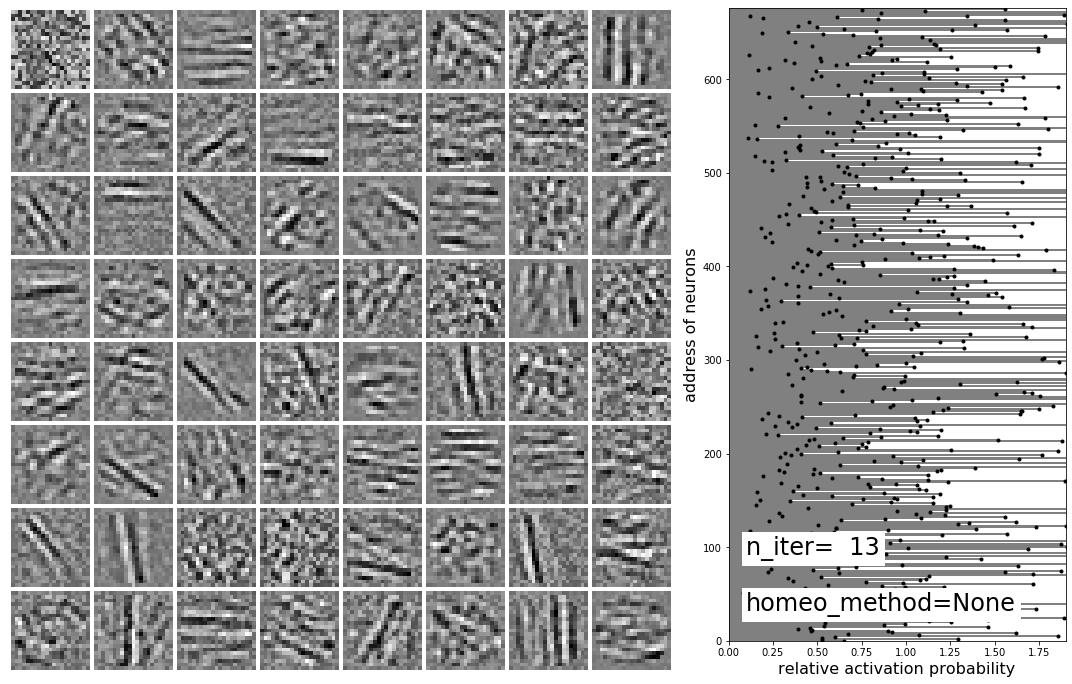```loading the dico called : /tmp/cache_dir/2019-09-11_Perrinet19_None_6_dico.pkl
==> i_iter=6 / n_iter=21
Coding data with algorithm  mp ```